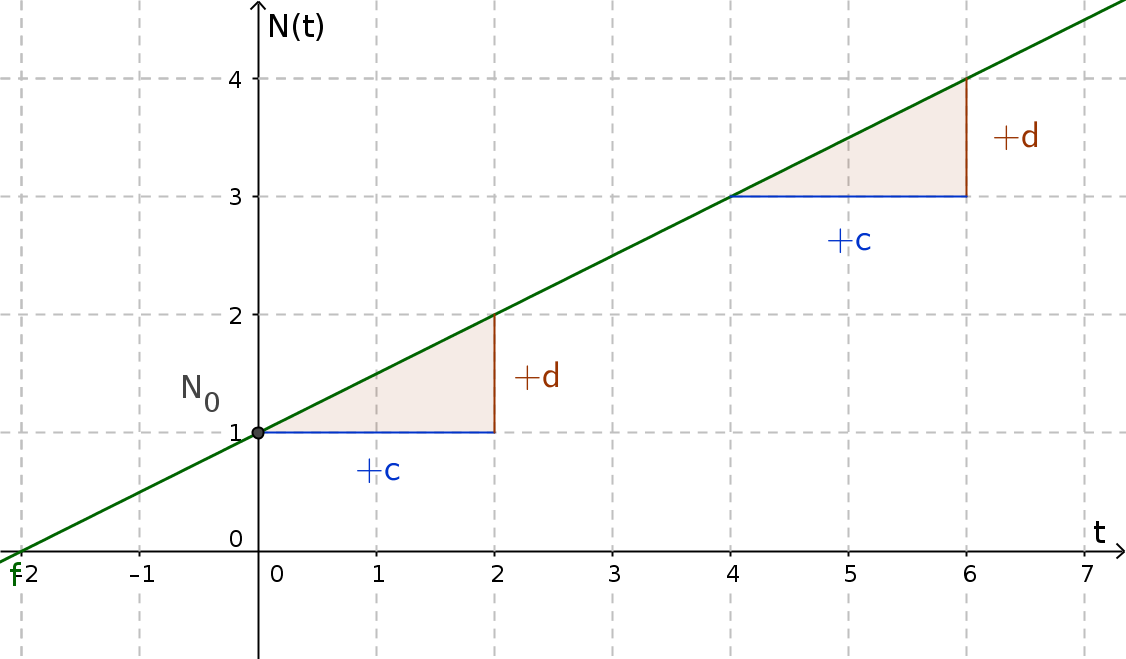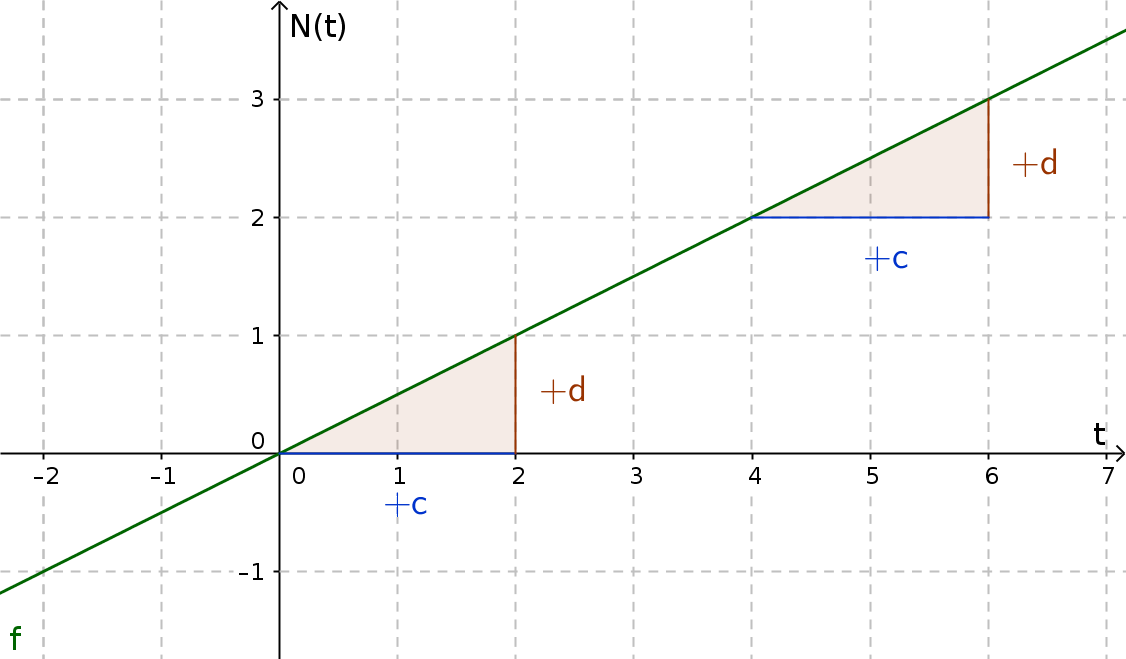Math Growth and decay Linear and proportional growth

# Linear and proportional growth

## Linear growth

In the case of linear growth, the value $N(t)$ for the same period of time ($c$) is always added with the same addend ($d$)The general equation of linear growth is:

$N(t)=m\cdot t + N_{0}$

$t...$ Period of time
$m ...$ Growth factor
$N(t) ...$ Value in dependence on $t$
$N_{0} ...$ Initial amount/start value

!

### Remember

Linear growth is described by linear functions.

## Proportional growth

Proportional growth is like linear growth, but with an initial amount of 0.The general equation would be:

$N(t)=m\cdot t$

$N_{0}$ falls away because the starting value is 0.